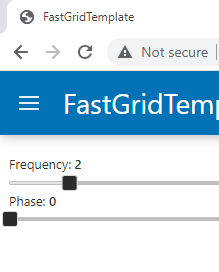# Favicon folder

Hi Everyone

Kindly please advise where is favicon folder so I can put favicon inside the folder since when I use this example:
import hvplot.pandas
import numpy as np
import panel as pn
import pandas as pd

xs = np.linspace(0, np.pi)

freq = pn.widgets.FloatSlider(name=“Frequency”, start=0, end=10, value=2)
phase = pn.widgets.FloatSlider(name=“Phase”, start=0, end=np.pi)

def sine(freq, phase):
return pd.DataFrame(dict(y=np.sin(xs*freq+phase)), index=xs)

def cosine(freq, phase):
return pd.DataFrame(dict(y=np.cos(xs*freq+phase)), index=xs)

dfi_sine = hvplot.bind(sine, freq, phase).interactive()
dfi_cosine = hvplot.bind(cosine, freq, phase).interactive()

plot_opts = dict(
responsive=True, min_height=400,
# Align the curves’ color with the template’s color
color=pn.template.FastGridTemplate.accent_base_color
)

# Instantiate the template with widgets displayed in the sidebar

template = pn.template.FastGridTemplate(
title=“FastGridTemplate”,
favicon=“images/favicon.ico”,
sidebar=[freq, phase],
)

# Populate the main area with plots, to demonstrate the grid-like API

template.main[:3, :6] = dfi_sine.hvplot(title=‘Sine’, **plot_opts).output()
template.main[:3, 6:] = dfi_cosine.hvplot(title=‘Cosine’, **plot_opts).output()
template.servable();

Favicon was not show up.

Images folder in myapp folder. Or may be I put wrongly the url for favicon

thanks